Courses

# Wave Guides and Resonating Cavities Electrical Engineering (EE) Notes | EduRev

## Electromagnetic Theory

Created by: Machine Experts

## Electrical Engineering (EE) : Wave Guides and Resonating Cavities Electrical Engineering (EE) Notes | EduRev

The document Wave Guides and Resonating Cavities Electrical Engineering (EE) Notes | EduRev is a part of the Electrical Engineering (EE) Course Electromagnetic Theory.
All you need of Electrical Engineering (EE) at this link: Electrical Engineering (EE)

Resonating Cavity

We have shown in the last lecture that the electric field components for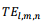are given by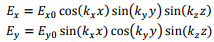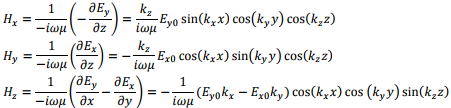Let us consider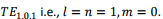This implies that ky = 0, leading to Ex=0 and Hy=0 Thus the only non-zero components are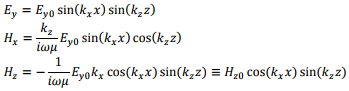where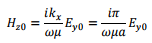In terms of Hz0 the fields are rewritten as,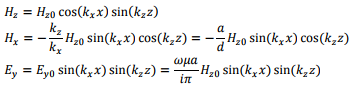Q- Factor of a Resonator

The Q-factor, a short form for Quality factor of a resonator is defined as the ratio of the amount of energy stored in the cavity and the amount of energy lost per cycle through the walls of the cavity. In the following we will calculate the Q factor for the TE1,0,1 mode of the cavity. The deterioration of the cavity is because of two factors, viz. the finite conductivity of the walls of the cavity and an imperfect dielectric in the space between. We will consider the space between the walls to be vacuum so that it is only the finite conductivity of the walls that we need to worry about. The conductivity, though not infinite, is nevertheless large so that the skin depth is small. The tangential component of the magnetic field will be assumed to be confined to a depth equal to the skin depth which leads to a surface current Js.

Let us first calculate the stored energy. The average energy stored is given by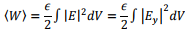since the only non-zero component of the electric field is along the y direction. Substituting the expression for Ey, we get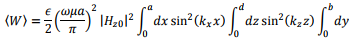The first two integrals respectively give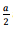and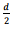while the last one gives b.We thus have,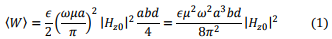We will now calculate the loss through the walls of the wave guide.

We know that the surface current causes a discontinuity in the magnetic field. As the magnetic field decrease fast within the skin depth region, we will assume the field to be zero in this region and calculate the surface current from the relation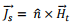where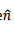is the unit normal pointing into the resonator.

For the rectangular parallelepiped we have three pairs of symmetric surfaces, contribution from each pair being the same. For instance we have a front face at x=α and a back face at x=0.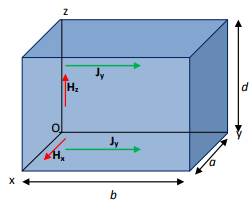For both the front face the normal direction is along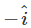and for the back face it is along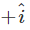since it is inward into the cavity. The surface current density is thus given by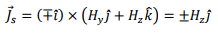since Hy=0 in this mode. We are, however interested in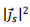so that contribution from the two walls add up and we get the loss from these two sides as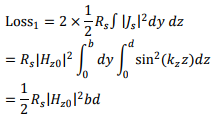we can in a similar way calculate the contribution from the left (y=0) and the right (y=b) faces for which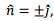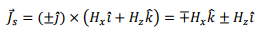so that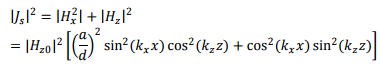Substituting this and integrating, the loss from the two side walls becomes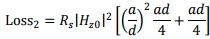In a similar way the loss from the top and the bottom faces can be found to be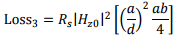Adding, all the losses, the total loss becomes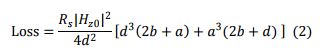The Q factor is given by ω times the quotient of [1) with (2)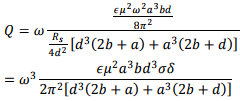where we have substituted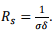thus the quality factor can be determined from a knowledge of the dimension of the cavity, the operating frequency and the nature of the material of the walls.

Circular Wave Guides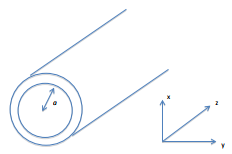The waveguide that we consider are those with circular cross section. The direction of propagation is still the z axis so that the geometry is cylindrical. This geometry is of great practical value as optical fibers used in communication have this geometry. However, optical fibres are dielectric wave guides whereas what we are going to discuss are essentially hollow metal tube of circular cross section.

We take the cylindrical coordinates to be (ρ,φ,z) and the radius of cross section to be a. We will first express the two curl equations in cylindrical.

As there are no real current, we have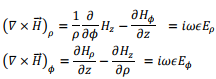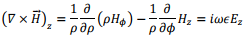As before, we will replace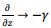so that these equations become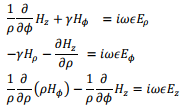The other set of the curl equations are obtained from Faraday’s law and can be easily written down from the above set by replacing ∈ with - μ and interchanging E and H.

We get,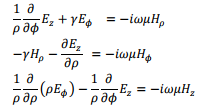As in the case of rectangular wave guides, we can still classify the modes as TE or TM. Consider one pair of the above equations,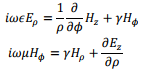we can eliminate Hφ from these two equations, and express Eρ as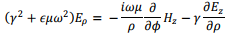Define, γ2 + ∈μω2= k2 to rewrite this equation as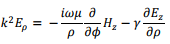Thus we have succeeded in expressing Eρ in terms of derivatives of the z component of E and H. In a similar way we can express all the four components.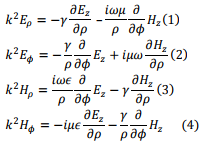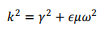We will now obtain solutions for Eand Hz using Helmholtz equation. In the following we will discuss the TE modes for which Ez =0 We need to then find Hz using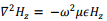Writing the Laplacian in cylindrical coordinates,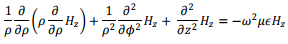We use technique of separation of variables by defining,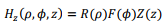Substituting this into the Helmholtz equation and dividing throughout by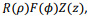we get,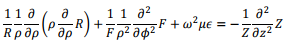The left hand side of this equation is a function of (ρ,φ) while the right hand side is a function of z alone. Thus we can equate each side to a constant. Anticipating propagation along the z direction, we equate the right hand side to -γ2  so that, we have,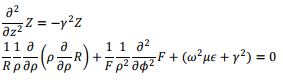The last equation needs to be separated into a term involving ρ alone and another depending on φ alone. This is done by multiplying the equation by ρ2,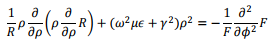Once again, we equate each of the terms to a constan n2. We thus have the following pair of equations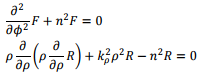where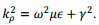The former equation has the solution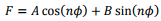Singlevaluedness of F requires that if φ changes by 2π the solution must remain the same. This, in turn,requires that n is an integer. We are now left with only the last equation which can be written in an expanded form,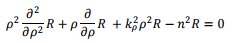Defining a new variable x=kρρ (not to be confused with the Cartesian variable x), the equation can be written as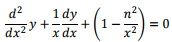where, we have written y in place of R. This equation is the well known Bessel equation, the solutions of which are linear combinations of Bessel functions of first kind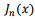and that of the second kind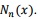The Bessel functions of second ind are also known as Neumann function. The following graph shows the variation of these functions with distance x.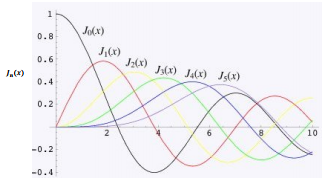It is seen that the Neumann function diverges at the origin and hence is not n acceptable solution. The asymptotic behavior of these functions are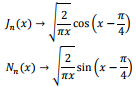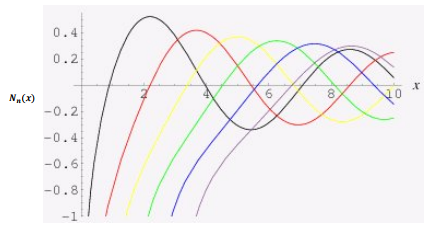The complete solution is thus given by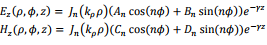For TE modes,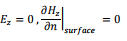For TM modes,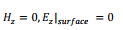Let us look at the TE mode in a little more detail. The tangential component of the electric field must be zero at the metallic boundary. Thus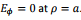Substituting the expression for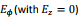we require, from Eqn. (2)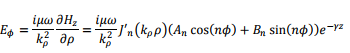where J'n is the derivative of Bessel function with respect to ρ. This expression vanishes at  ρ=α provided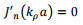which provides a restriction on propagation of TE mode. The zeros of the Bessel function Jare listed in the following table, (m-th zero of Jρ nm )

 n m=1 m=2 m=3 0 2.4048 5.5201 8.6537 1 3.8317 7.0156 10.1735 2 5.1356 8.4172 11.6198

The zeros of the derivative of the Bessel function are listed in the following table (m-th zero of Jρ nm )

 n m=1 m=2 m=3 0 3.8317 7.0156 10.1735 1 1.8412 5.3314 8.5363 2 3.0542 6.7061 9.9695

For propagation to take place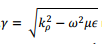must be imaginary. This is possible if ω>ωc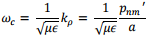where ρnm'  gives the m-thzero of the derivative of Bessel function. The corresponding mode is classified as TEnm Similarly, one can show that the cutoff frequency for the TM modes are given by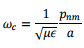From the tables given above, it is clear that the lowest mode is TE11 followed by TM01

The field pattern of the waveguide are shown in the following (source – Web)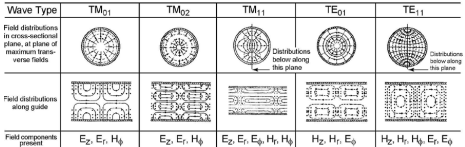Tutorial Assignment

1. TE10 mode propagates in an air filled rectangular waveguide of dimension 1cm x 0.5cm. The frequency of the propagating wave is 3x 1012 Hz. It is desired to construct a cavity out of this waveguide by closing the third dimension so that the cavity resonates in TE102 mode. (a) What should be the length of the cavity? (b) Write down the electric field for the resonating mode.
2. For a cubical cavity, what is the degeneracy of the lowest resonant frequency, i.e. how many different modes correspond to the lowest possible frequency?
3. A circular (cylindrical) waveguide of radius 4 cm is filled with a material of dielectric constant 2.25. The guide is operated at a frequency of 2 GHz. For the dominant TE mode, determine the cutoff frequency, the guide wavelength and the bandwidth for a single mode operation (assuming only TE modes).
4. If the cylindrical cavity of circular cross section is closed at both ends by perfectly conducting discs, it becomes a cavity resonator. Find the resonant frequencies of a cavity resonator of length d and radius a.

Solutions to Tutorial Assignments

1. For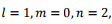the resonant frequency is given by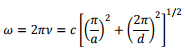Substituting α=0.01, and the value of the frequency, we get, d = 1.15 cm. With these values,we have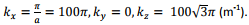The non-vanishing field components are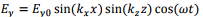The other two non-vanishing field components can be calculated by using Maxwell’s equations,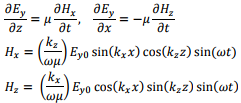2. Since all the three dimensions are the same, we would have the same frequency for (100), (010) and (001) modes. Counting TE and TM, there are 6 modes corresponding to the lowest frequency.

3. For TEmn mode the cutoff frequency is given by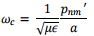For the dominant mode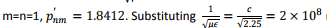m/s, and a=0.04 m, we getωc= 9.206 � 109 rad/s corresponding to 1.47 GHz. To find the guide wavelength, note that the propagation vector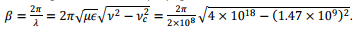Solving, we get λ = 0.14 m. To find the bandwidth of single mode TE operation note that the since the cutoff frequency for the next higher mode TE21 is 3.0542/1.8412=1.66 times the cutoff frequency for TE11 mode, the bandwidth is fixed.

4. If the cavity resonator is closed at both ends, there would be standing waves formed along the z direction. Since the tangential component of the electric field is continuous (and therefore vanishes near the surface of the perfect conductor,) we must have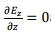at z=0 and z=d.

Taking the origin at the centre of one of the disks, for TM mode, Ez is given by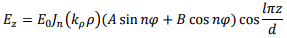Since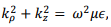the resonant frequencies for the TM modes are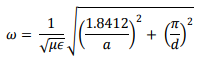Note that unlike the dominant frequency of the TM mode which is fixed by the dimension a, the TE111 frequency can be tuned by adjusting the value of the length of the resonator. If d is made sufficiently large, the frequency can be made to be lower than that of the TM mode.

Self Assessment Questions

1. Consider TE011 mode in a cubical cavity of side a. Write the expressions for the electric and magnetic fields and show that they are orthogonal.
2. For the above problem determine the total energy stored in the electric and magnetic fields.
3. A cubical cavity of side 3 cm has copper walls (conductivity σ= 6 � 107 S/m). Calculate the Q factor of the cavity for TE101 mode.
4. Consider a cylindrical cavity resonator. Show that the dominant frequency is TE mode of the resonator.

Solutions to Self Assessment Questions

1. By definition of TE mode, Ez=0. Further, for 011 modes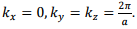This gives Ey=0.We have,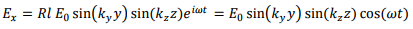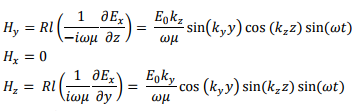2. Total energy in the electric field is obtained by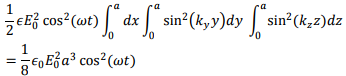One can similarly find the magnetic field energy from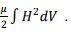This gives,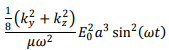Using the relation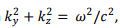this expression can be written as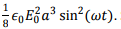So that the total energy in the field is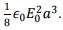3. The resonant frequency of the cavity for TE101 mode is given by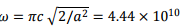rad/s. Using the expression given in the text, the Q factor can be written as follows for the caseof α=b=c.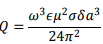Equivalent expressions for Q factor can be written using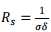and the preceding expression for ω as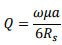Where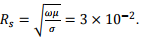Substituting these values we get Q ≈ 9300.

4. For the TE mode of the cavity Bz must vanish at the surface of the end faces. The z –component of the magnetic field is, therefore, given by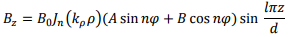The resonant frequencies are given by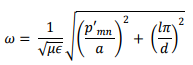where ρmn are the zeros of the derivatives of Bessel functions. Note that unlike in the case of TM modes, in this case ℓ≠0 because that would make the fields vanish. For TM modes, the dominant frequency is TM010, for which the frequency is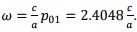The dominant mode for TE is TE111 for which the frequency is given by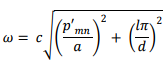,

,

,

,

,

,

,

,

,

,

,

,

,

,

,

,

,

,

,

,

,

;